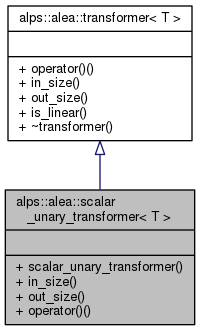ALPSCore reference
alps::alea::scalar_unary_transformer< T > Struct Template Reference

#include <transformer.hpp>

Inheritance diagram for alps::alea::scalar_unary_transformer< T >:Collaboration diagram for alps::alea::scalar_unary_transformer< T >:## Public Member Functions

scalar_unary_transformer (const std::function< T(T)> &fn)

size_t in_size () const

size_t out_size () const

column< T > operator() (const column< T > &in) constPublic Member Functions inherited from alps::alea::transformer< T >
virtual bool is_linear () const

virtual ~transformer ()

## Detailed Description

### template<typename T> struct alps::alea::scalar_unary_transformer< T >

Definition at line 19 of file transformer.hpp.

## Constructor & Destructor Documentation

template<typename T >
 alps::alea::scalar_unary_transformer< T >::scalar_unary_transformer ( const std::function< T(T)> & fn )
inline

Definition at line 74 of file transformer.hpp.

## Member Function Documentation

template<typename T >
 size_t alps::alea::scalar_unary_transformer< T >::in_size ( ) const
inlinevirtual

expected number of components of the input vector

Implements alps::alea::transformer< T >.

Definition at line 76 of file transformer.hpp.

template<typename T >
 column alps::alea::scalar_unary_transformer< T >::operator() ( const column< T > & in ) const
inlinevirtual

apply transformation

Implements alps::alea::transformer< T >.

Definition at line 80 of file transformer.hpp.

template<typename T >
 size_t alps::alea::scalar_unary_transformer< T >::out_size ( ) const
inlinevirtual

number of components of the returned vector

Implements alps::alea::transformer< T >.

Definition at line 78 of file transformer.hpp.

The documentation for this struct was generated from the following file: